# Egg tossing crime -- From what location was the egg thrown?

• Aion96
In summary, from the conversation it can be concluded that a map of the crime scene is needed to determine the possible throwing areas. The throw length must be within the intervals of 11-43 meters, and the throw height must be within the range of 0-12.5 meters. The starting speed of the thrown object can be calculated using formulas that take into account the distance and height from which it was thrown. Excel can be used to determine the required starting speed for different values of distance and height.

#### Aion96

Homework Statement
Fermi problem.

During the winter season, a few of my neighbour had eggs thrown at them as they passed the street. Each attack occurred at the same place (see the red dot on the map) but with no apparent individuals in the vicinity. The egg thrower is tossing the eggs from indoors och probably from some of the windows marked as green on the map. The area painted red designates where the egg cannot have been thrown since there are no openable windows and dense vegetation in the way. The houses number 5 has three floors and the houses 14/16 has 4 floors and are also stationed 2,5m above the road. Figure 1 is to the given scale.

From what location can the culprit have thrown the egg? Since there will be many variables, assume that it is children between the age 8 and 16 who can throw the egg and that they do so at a 45 ° angle. One floor is about 2.5m high.
Relevant Equations
Herons Formula ##A=\sqrt{p(p-a)(p-b)(p-c)}, \quad p= a+b+c ##

Kinematics formulas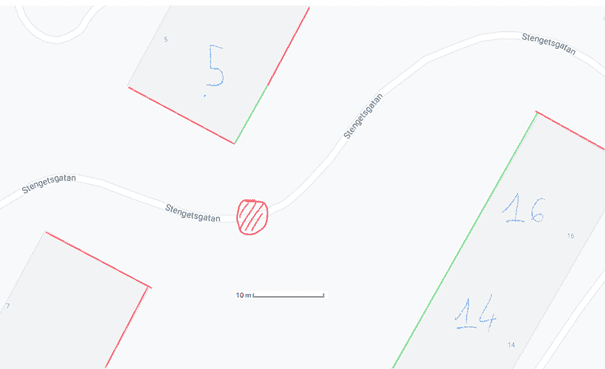Figure 1. A map of the crime scene.

My attempt:

We start by trying to map the possible throwing area from the green marked walls in the figure. The area can be divided into two suitable triangles from each corner towards the red marked crime scene. With the help of Google Earth, the sides of the triangles can be approximated quite well.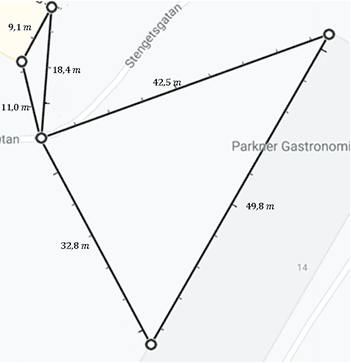Figure 2. The throw area is divided into two parts.​

Observe from the figure that the throw length d must be limited to the interval.

$$11 \space m\leq d\leq 43 \space m \quad(1)$$

Also, note that the throw length from house number 5 is within the range

$$11 \space m \leq d \leq 18 \space m \quad (2)$$

We round off a bit to simplify the calculations somewhat. It is a little more complicated to determine the minimum throw length to house number 14/16. According to Pythagoras' theorem, the shortest distance between two points is the perpendicular distance. So we search for the height of the large triangle. One can calculate the height using Heron's formula. The formula gives the area ##A## for a triangle expressed by the side lengths ##a,b,c## as

$$A=\sqrt{p(p-a)(p-b)(p-c)}, \quad p= a+b+c \quad (3)$$

Where ##p## is the circumference of the triangle. Now we define it according to figure 2.

$$a=32.8 \space m,b=49.8 \space m,c=42.5 \space m \quad (4)$$

Then the half circumference is calculated as

$$p=\frac{a+b+c}{2}=62.55 m (5)$$

Then the area of the triangle is calculated according to Heron's formula.

$$A=\sqrt{p(p-a)(p-b)(p-c)}\approx 689.7146 \space m^2 \quad (6)$$

Using the second area formula, the height of the triangle can be calculated

$$A=\frac{bh}{2} \quad (7)$$

$$h=\frac{2A}{b}=27.699 m \approx 28 \space m \quad (8)$$

Hence the shortest distance from the crime scene to house 14/16 is approximately ##28 \space m##. Now it is obvious ´from figure 2 that the horizontal throw range ##d## for house number 14/16 follows the condition.

$$28 \space m\leq d \leq 43 \space m \quad (9)$$

The throw height can also be limited within a range if it is assumed that a floor is ##2.5 \space m##. House number 14/16 will give the maximum height. These houses consist of four floors and are located about ##2.5 \space m## above the ground. The maximum throw height is therefore approximately ##12.5 \space m##, which means that the start height for the thrown egg is within the range.

$$0 \space m\leq h \leq 12.5 \space m \quad (10)$$

But it is important to point out that the height will always consist of discrete quantities. Each possible height is always the product of the number of floors ##n## with the height of each floor. The amount of solution is therefore given by:

$$h=2.5n \quad (11)$$
Where n is an integer between 0 and 5. Note that there is a maximum of 4 floors, but house number 14/16 is also ##2.5 \space m## above the ground. To arrive at a formula for the egg's starting speed, air resistance is neglected. The launch velocity of the projectile is calculated by treating the movement in the ##x## and ##y## directions separately. For the movement in the ##x-##direction, the projectile has a constant speed.

$$v_{x}=v_{x_{i}}=v_{i}\cos(\alpha) \quad (12)$$

$$x=v_{x_{i}}t+x_{i}=v_{i}\cos(\alpha)t+x_{i} \quad (13)$$

For the movement in the y-direction, the projectile has a constant acceleration with the negative acceleration ##−g##.

$$v_{y}=-gt+v_{y_{i}}=-gt+v_{i}\sin(\alpha) \quad (14)$$

$$y=-\frac{1}{2}gt^2+v_{y_{i}}t+h=-\frac{1}{2}gt^2+v_{i}\sin(\alpha)+h \quad (15)$$

The starting conditions are.

$$x_{i}=0, h\ge 0, \quad (16)$$

And the final conditions when the projectile reaches the ground.
$$t=T, x=d, y=0, \quad (17)$$

If you combine the final conditions with equations (13) and (15), you get two equations with the two unknown variables ##T## and ##v_{i}##. If ##T## is eliminated you get an expression for ##v_{i}##.

$$v_{i}=\frac{1}{\cos(\alpha)}\sqrt{\frac{0.5gd^{2}}{d\tan(\alpha)+h}} \quad (18)$$

Assuming that the throw angle is 45°, the formula for the initial velocity is simplified.

$$v_{i}=\sqrt{\frac{gd^2}{d+h}} \quad (19)$$

Where ##d## is the distance in the x-direction and ##h## the initial height. The throwing speed in formula (19) depends partly on the height from which the egg was thrown, and partly on the horizontal distance from the crime scene. We will now calculate the starting speed ##v_{i}## for a number of values for ##d## and ##h## within each interval. We make these calculations using Excell to try and identify what the starting speed may be from each house.

Table 1. Each height and velocity is calculated for various values of ##n##. The blue marked part of the table refers to house number 5 for ##d## within the range ##11≤d≤18##. We count from the ground floor of the house to the third floor and are therefore only interested in values of n from zero to 3. The yellow marked part of the table refers to the houses 14/16 where the throw length is within the range ##28≤d≤43##, where we are interested in values on n from 1 to 5.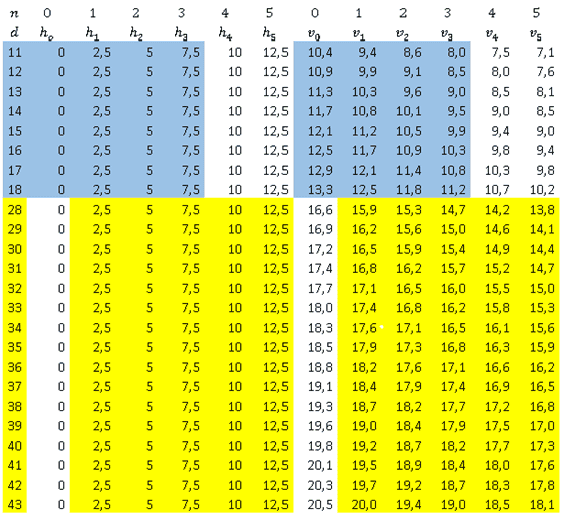Note from the table that the distance d affects the required starting speed the most. Since building 14/16 is located further away from the crime scene, these houses require a greater initial velocity . The height does not affect the initial velocity as much, but a larger height decreases the required velocity. By categorizing velocities the from the table above in order of magnitude, one find that they are limited to the following intervals.

$$8 \space m/s \leq v_{i}\leq 20 \space m/s \quad (20)$$

The eggs start velocity from house number 5 are within range

$$8 \space m/s \leq v_{i}\leq 13.3 \space m/s \quad (21)$$

And the start velocity from house number 14/16 within interval

$$13.8\space m/s \leq v_{i}\leq 20\space m/s \quad (22)$$

There is no unambiguous start velocity for the egg. But one can assume that individuals between the ages of 8 and 16 cannot throw the egg at a greater velocity than a professional pitcher in softball. According to a source, individuals in this age group throw with start velocities between 15−25m/s (Anon., 2021) Assuming that the individual throws the egg with full force, this interval is closer to house number 14/16. It is reasonable that the egg was thrown from house 14/16. But this conclusion is not very well based as eggs have a different mass, surface area and strength than the ball that is thrown at softball. However, there are other reasons for drawing this conclusion. For these houses have more floors and at the same time extend over a larger area. Therefore, the houses accommodate more individuals who may have thrown the egg.

If there was a maximum throwing speed from which the egg could be thrown without breaking, the maximum speed could be used to exclude the speed in the table that exceeded this maximum value. The world record in egg throwing seems to be ##99.51 \space m##. The average value for a man's height in the USA is about ##1.77 \space m##. The maximum horizontal range occurs approximately at a throw angle of 45 ° when neglecting air resistance. With these values, the initial velocity for the world record becomes approximately.

$$v_{i}=\sqrt{\frac{gd^2}{d+h}} \approx 31 \space m/s \quad (23)$$

Unfortunately, this value is far above the start velocity in the table and therefore no velocity can be ruled out. There does not seem to be a satisfactory answer as to where the egg may have been thrown from. All houses have possible places from which the egg can be thrown with existing information. In any case, we have arrived at the speed ranges within which the egg must be limited from each house number. If the initial speed had been known, these could have been used to identify where the egg was thrown from. The method used can certainly be improved by dividing d into smaller parts and by avoiding roundings. In this model, we also did not take into account the person's height, but counted from the floor level from each floor. But if you add the height of the person, the height is not much affected. A further shortcoming with our model is the throw angle is a variable and not necessarily 45 °.

Is it possible that the throwing speed is normally distributed depending on age? The normal distribution of eggs throwing speeds is unfortunately not easily accessible information on the internet. If there was such a normal distribution, one could possibly use the standard deviation and the mean value to calculate the probability that the egg was thrown from each house. I am very intressted if the reader have any sugguestions as to how one could tackle this problem from another perspective!

Sincerely Aion

Last edited:
Welcome to PhysicsForums.

Wow, that is a detailed and involved homework problem! BTW, what do the roofs of these buildings look like? Do any of them have flat roofs with roof access?

berkeman said:
Welcome to PhysicsForums.

Wow, that is a detailed and involved homework problem! BTW, what do the roofs of these buildings look like? Do any of them have flat roofs with roof access?
Thank's. I normally don't spend this much time on one problem. I am not satisfied with my conclusions, although I did my best anyway. The coordinates for the location at google Earth is 57.679459, 11.883131. These buildings does not appear to have any roof access.

Aion96 said:
The coordinates for the location at google Earth is 57.679459, 11.883131. These buildings does not appear to have any roof access.
The buildings above and to the lower left appear to have flat roofs, and almost certainly have roof access. Maybe some kids figured out how to get up there, and played "sniper" with the eggs...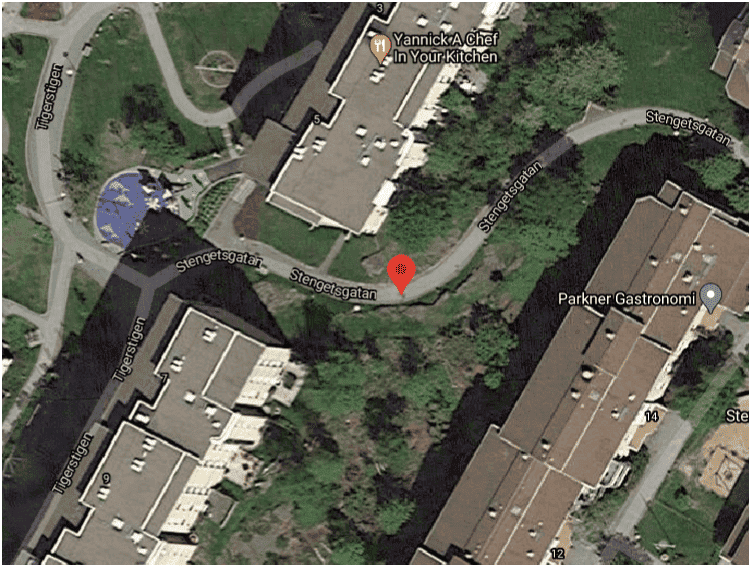•Aion96
berkeman said:
The buildings above and to the lower left appear to have flat roofs, and almost certainly have roof access. Maybe some kids figured out how to get up there, and played "sniper" with the eggs...

View attachment 281609
Haha I didn't notice that. That's actually a real possibility. Thank you for your insight, it was very helpful.

•berkeman
Maybe you'll get extra credit for the innovative answer.Also, you may be able to quantify how much easier it is to toss the offending eggs from the roofs versus having to fling them out a lower window...

berkeman said:
Also, you may be able to quantify how much easier it is to toss the offending eggs from the roofs versus having to fling them out a lower window...
Do mean by tossing eggs from a given height and compare it with the work required for tossing it from ground level? That sounds reasonably simple. I think I need a comparable building to throw the eggs from since I don't live at this location. Hopefully, I won't hit anyone with my eggs.

Last edited:
•berkeman
One can fashion a sling made of, say, elastic material of the kind that people use for exercise and attach its ends to either side of the open window. That will enhance the range and accuracy of the projectiles considerably. Have you considered that?

Also, I noticed a fair amount of what looks like trees or perhaps bushes around the crime scene. Is it possible to exclude some windows because they don't provide a clear shot to the target? Moreover, to catch a thief you must think like one. What window would you use if you were the perpetrator? Finally, if all else fails, install a couple of surveillance cameras.

•Aion96
Aion96 said:
Homework Statement:: Fermi problem.

All houses have possible places from which the egg can be thrown with existing information
I think the key is that the eggs land in one spot and only one spot.

From certain windows, the line of sight of someone visible on the street has a wide range, and eggs could be tossed to land within this wide range minus the distance traveled by the vehicle or walking person when first seen to the time of egg splat, which would be the velocity of the person times the time of flight of the egg ( is it only a walkway perhaps - 2m/sec or for a slow car 10 to15m/sec ). For some other windows, the range is much narrower.

Take the top building.
The person/car can be seen only when passing by the corner of the building from one direction, or when passing by the trees from the other direction. The distance from these locations to the egg splat is about 11 meters. Assuming a 1 sec ready-aim-fire and a 3 sec time of flight ( assuming time of flight you will have to calculate ) a person moves only 8 m walking and a slow car moves 40 m - maybe the cars are moving slower.

For the right hand building, the person/car would be noticed passing by the corner of the right hand building or the corner of the bottom building depending upon direction. Using the same ready-aim-fire and time of flight, a car could traverse what looks like 40 meters from either direction to the splat in the time allocated.

To find the actual window, one has to refine the ready-aim-fire timelength ( assuming it is identical for both directions of car/person ie symmetry ) and the time of flight ( also symmetric ) from a window of a particular height from either building.

•Aion96
kuruman said:
One can fashion a sling made of, say, elastic material of the kind that people use for exercise and attach its ends to either side of the open window. That will enhance the range and accuracy of the projectiles considerably.
Um, it kind of sounds like you have some experience with this method...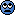berkeman said:
Um, it kind of sounds like you have some experience with this method...An accurate guess but the experience is second-hand. When I was in college I knew someone whose father was a physician. He "borrowed" some tourniquet-grade stuff from dad and fashioned a sling which he said he used to launch oranges into a lacrosse field when nobody was there. I never saw him do it but I saw the sling. I thought the whole idea was hilarious, but then a lot of things seemed hilarious to me at the time.

•Aion96 and berkeman
IF this was a real-world problem, all that's required is to look at the splatter pattern.•Aion96
256bits said:
I think the key is that the eggs land in one spot and only one spot.

From certain windows, the line of sight of someone visible on the street has a wide range, and eggs could be tossed to land within this wide range minus the distance traveled by the vehicle or walking person when first seen to the time of egg splat, which would be the velocity of the person times the time of flight of the egg ( is it only a walkway perhaps - 2m/sec or for a slow car 10 to15m/sec ). For some other windows, the range is much narrower.

Take the top building.
The person/car can be seen only when passing by the corner of the building from one direction, or when passing by the trees from the other direction. The distance from these locations to the egg splat is about 11 meters. Assuming a 1 sec ready-aim-fire and a 3 sec time of flight ( assuming time of flight you will have to calculate ) a person moves only 8 m walking and a slow car moves 40 m - maybe the cars are moving slower.

For the right hand building, the person/car would be noticed passing by the corner of the right hand building or the corner of the bottom building depending upon direction. Using the same ready-aim-fire and time of flight, a car could traverse what looks like 40 meters from either direction to the splat in the time allocated.

To find the actual window, one has to refine the ready-aim-fire timelength ( assuming it is identical for both directions of car/person ie symmetry ) and the time of flight ( also symmetric ) from a window of a particular height from either building.
Wow. I'll try your metod and see what I find.

catapult on a rooftop, disguised as a deck-chair ; spotter on a porch, orthogonal to projectile path.

(nevermind, found two probable launch points)

Last edited: